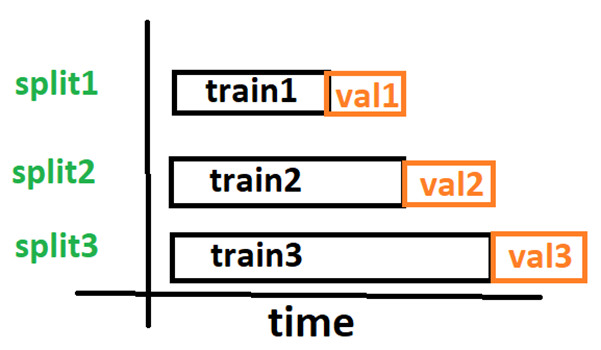# Walk forward cross-validation with Optuna and deepar in pytorch forecasting

I want to perform 3 splits walk forward cross validation with expanding training set for the deepar model from the pytorch forecasting framework. When I do walk forward validation, I also want to do hyperparameter optimization using Optuna.

Currently, the setup is normal validation (i.e. split1 only). After every epoch, the validation loss is calculated and passed to Optuna which uses it to prune the trial if necessary. A trial is one of the many possible combinations of hyperparameters. This is a kind of early stopping done by Optuna to prevent training of further epochs in that trial to save time.

If my understating of walk forward cross validation is correct, I would like to use 3 folds of validation that are temporally increasing. The picture below shows the splits.For every epoch, I would like to synchronously perform trainer.fit() for all the three splits and then get their average validation loss after each epoch and pass it to Optuna to decide whether to prune this trial or not. Do I have to do some kind of multithreading or is there any setting in deepar that can achieve this?

Is there another way to perform walk forward validation? I mean like doing sequentially for each split at a time. So first for split1 many epochs are tried until early stopping condition is met (i.e. validation loss does not improve). Next split2 is trained and validated like split1 to obtain minimum validation loss for split2. The same happens with split3. Finally all three minimum validation loss are averaged. Note that all 3 splits might have stopped at different epochs but we just ignore that and only take the minimum validation loss in each split. In this case how to use Optuna to prune the trial?

Currently, the objective function in Optuna looks like below:

``````def objective(trial,):

neu = trial.suggest_int(name="neu",low=600,high=800,step=25,log=False)
lay = trial.suggest_int(name="lay",low=1,high=3,step=1,log=False)
bat = trial.suggest_int(name="bat",low=4,high=12,step=4,log=False)
lr = trial.suggest_float(name="lr",low=0.000001,high=0.01,log=True)
num_ep = trial.suggest_int(name="num_ep",low=20,high=30,step=2,log=False)
enc_len = encoder_length
pred_len = 1
drop = trial.suggest_float(name="dropout",low=0,high=0.4,step=0.1,log=False)

train_dataset = TimeSeriesDataSet(
train_data,
time_idx="time_idx",
target=Target,
categorical_encoders=cat_dict,
group_ids=["group"],
min_encoder_length=enc_len,
max_encoder_length=enc_len,
min_prediction_length=pred_len,
max_prediction_length=pred_len,
time_varying_unknown_reals=[Target],
time_varying_known_reals=num_cols_list,
time_varying_known_categoricals=cat_list,
randomize_length=False,
scalers={},
target_normalizer=TorchNormalizer(method="identity",center=False,transformation=None )

)

val_dataset = TimeSeriesDataSet.from_dataset(train_dataset,val_data, stop_randomization=True, predict=False)

"""
Machine Learning predictions START
1) DeepAR

"""

metrics_callback = MetricsCallback()

trainer = pl.Trainer(
max_epochs=num_ep,
gpus=-1, #-1
auto_lr_find=False,
limit_train_batches=1.0,
limit_val_batches=1.0,
logger=True,
val_check_interval=1.0,
callbacks=[lr_logger,metrics_callback]
)

#print(f"training routing:\n \n {trainer}")
deepar = DeepAR.from_dataset(
train_dataset,
learning_rate=lr,
hidden_size=neu,
rnn_layers=lay,
dropout=drop,
loss=Loss,
log_interval=20,
log_val_interval=6,
# reduce_on_plateau_patience=3,
)

trainer.fit(
deepar,
)

metrics_list = [ metrics["val_RMSE"].item()  for metrics in  metrics_callback.metrics[1:]]
min_val_rmse = metrics_list[-1]

trial.report(min_val_rmse)

# Handle pruning based on the intermediate value.
if trial.should_prune():
raise optuna.exceptions.TrialPruned()

return min_val_rmse

``````